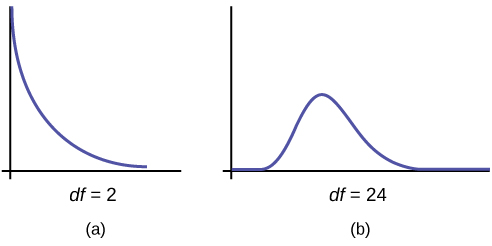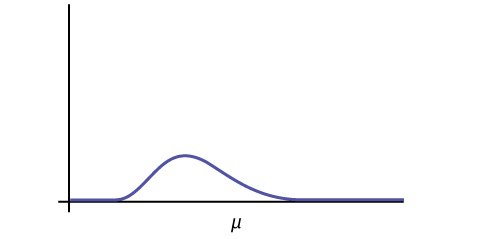## Facts About the Chi-Square Distribution

### Learning Outcomes

• Interpret the chi-square probability distribution as the sample size changes

The notation for the chi-square distribution is $\displaystyle\chi\sim\chi^2_{df}$, where df = degrees of freedom which depends on how chi-square is being used. (If you want to practice calculating chi-square probabilities then use $\displaystyle{df}=n-1$. The degrees of freedom for the three major uses are each calculated differently.)

For the χ2 distribution, the population mean is μ = df and the population standard deviation is $\displaystyle\sigma_{\chi^2}=\sqrt{2(df)}$.

The random variable is shown as χ2, but may be any upper case letter.

The random variable for a chi-square distribution with k degrees of freedom is the sum of k independent, squared standard normal variables.

$\displaystyle\chi^2=(Z_1)^2+(Z_2)^2+\dots+(Z_k)^2$

1. The curve is nonsymmetrical and skewed to the right.
2. There is a different chi-square curve for each df.3. The test statistic for any test is always greater than or equal to zero.
4. When df > 90, the chi-square curve approximates the normal distribution. For $\displaystyle{X}\sim\chi^2_{1,000}$ the mean, $\displaystyle\mu=df=1,000$ and the standard deviation, $\displaystyle\sigma=\sqrt{2(1,000)}$. Therefore, $\displaystyle{X}\sim{N}(1,000, 44.7)$, approximately.
5. The mean, μ, is located just to the right of the peak.## References

“HIV/AIDS Epidemiology Santa Clara County.”Santa Clara County Public Health Department, May 2011.

## Concept Review

The chi-square distribution is a useful tool for assessment in a series of problem categories. These problem categories include primarily (i) whether a data set fits a particular distribution, (ii) whether the distributions of two populations are the same, (iii) whether two events might be independent, and (iv) whether there is a different variability than expected within a population.

An important parameter in a chi-square distribution is the degrees of freedom df in a given problem. The random variable in the chi-square distribution is the sum of squares of df standard normal variables, which must be independent. The key characteristics of the chi-square distribution also depend directly on the degrees of freedom.

The chi-square distribution curve is skewed to the right, and its shape depends on the degrees of freedom df. For df > 90, the curve approximates the normal distribution. Test statistics based on the chi-square distribution are always greater than or equal to zero. Such application tests are almost always right-tailed tests.

## Formula Review

$\displaystyle\chi^2=(Z_1)^2+(Z_2)^2+\dots(Z_{df})^2$ chi-square distribution random variable

$\displaystyle\mu_{\chi^2}=df$ chi-square distribution population mean

$\displaystyle\sigma_{\chi^2}=\sqrt{2(df)}$ Chi-Square distribution population standard deviation